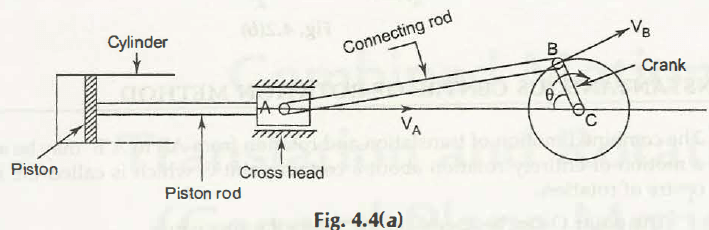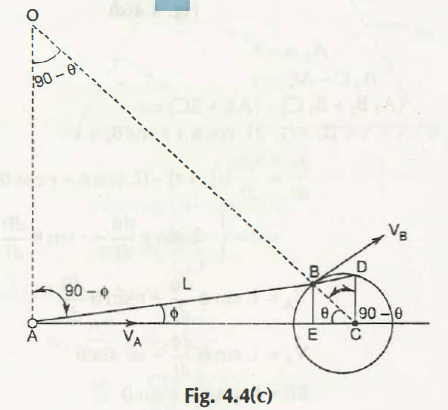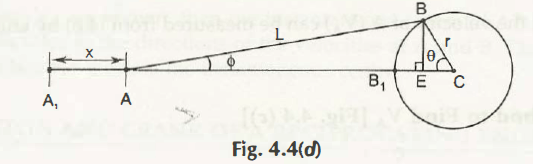Different parts of a reciprocating engine is shown in Fig. 4.4 (a)Let us consider this figure,

Point A will have linear motion towards point C along the line AC

Point B will have circular motion with an angular velocity

Thus,

Velocity at point A, VA = 0 AO

Similarly,

Velocity at point B, VB = C0 BO

Or,

r = 0 BO. 0r/ BO

VA = 0 AO

= (r) AO/ BO

Hence,

We can easily get the velocity at point A by knowing the values of all elements.

Analytical Method to find VAFig. 4.4 (c) shows the graphical representation of different parts of an engine.

To find the velocity at point A, we can use different analytical methods.

Method 1:

Draw line CD ⏊ AC and line BE ⏊ AC

Now,

In AOB and BCD

⦟AOB = 900 –  = ⦟BCD

⦟OAB = 900 –  = ⦟BDC     (we know that ⦟OBA = ⦟LCB)

Hence,

AO/ BO = CD/ BC = CD/ r

And,

tan = CD/ AC

CD = AC. tan

= (AE + EC) tan

= (L cos + r cos ) tan

= L sin  + r cos tan

Since,

VA = (r) AO/ BO

= (r) CD/ r

=  (L sin  + r cos  tan)

Method 2:

Suppose the crank is at IDC (Inner Death Centre). In this condition,

A1B1 = AB = L

B1C = r

Hence,

AO/ BO = CD/ BC = CD/ r

tan = CD/ AC

we know that

CD = AC. tan

= (AE + EC) tan

= (L cos + r cos ) tan

= L sin  + r cos  tan

Thus,

VA = (r) AO/ BO

= (r) CD/ r

=  (L sin  + r cos  tan)

Method 3:

Considering the Fig.4.4 (d),Let, A1A be x

It can be written as,

A1C – AC = x

(A1B1 + B1C) – (AE + EC) = x

(L + r) – (L cos + r cos ) = x

Differentiating the above equation with respect to time, we get-

=

= L sin+ r sin

Hence,

VA = L sin+ r sin

Since,

BE = L sin = r sin

sin = r/ L sin

Differentiating the above equation with respect to time, we get-

cos =r/ L

= r/ L cos

= rcos / Lcos

Now, substitute this value to get VA,

Therefore velocity at point A,

VA = L sin(rcos / Lcos ) + r sin

= r cos sincos  + r sin

=  (L sin  + r cos  tan)

Links of Next Mechanical Engineering Topics:-### Customer Reviews

My Homework Help
Rated 5.0 out of 5 based on 510 customer reviews at